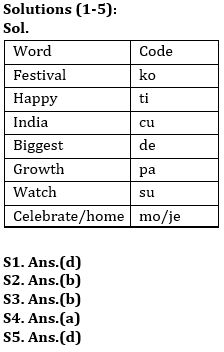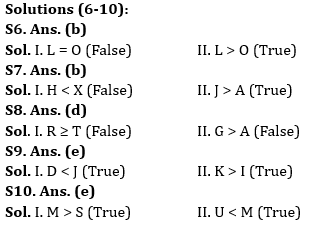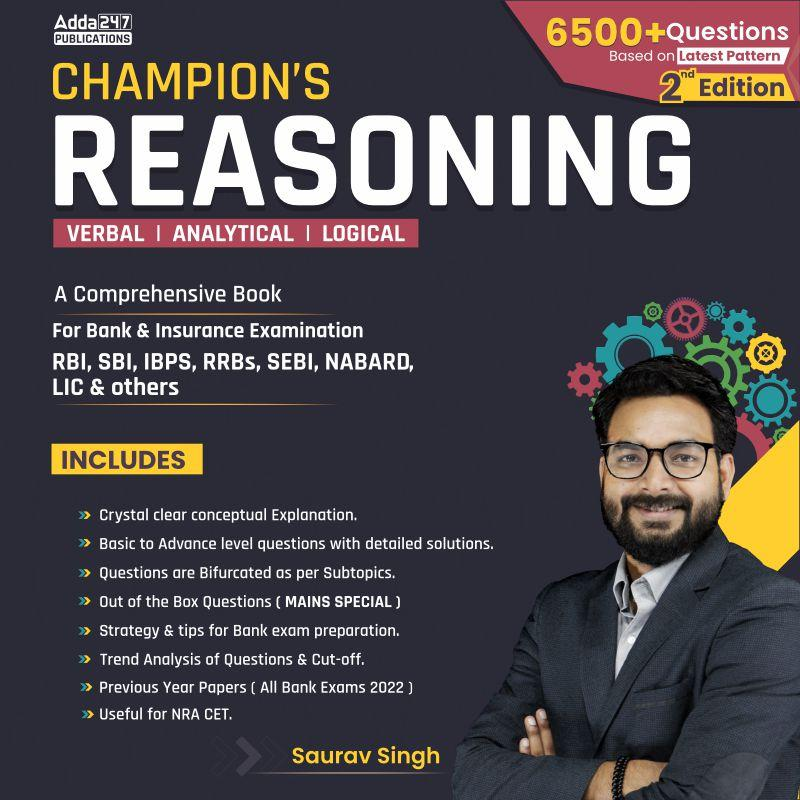Latest Banking jobs   »   IBPS reasoning

# Reasoning Quiz For IBPS PO Prelims 2023-08th September

Directions (1-5): Study the following information carefully to answer the given questions.
In a certain code language,
‘Festival celebrate happy home India’ is written as ‘ti cu ko mo je’,
‘India Biggest festival’ is written as ‘de cu ko’,
‘Biggest growth India’ is written as ‘cu pa de’
‘Festival happy watch India’ is written as ‘cu ti ko su’.

Q1. Which of the following is the code of word ‘home’?
(a) je
(b) ti
(c) mo
(d) Either (a) or (c)
(e) None of these

Q2. What does ‘ti’ stand for?
(a) Festival
(b) Happy
(c) Home
(d) Watch
(e) Can’t be determined

Q3. Which of the following will be the code for ‘India watch festival’?
(a) su cu ti
(b) su ko cu
(c) ti ko cu
(d) ko mo su
(e) None of these

Q4. Which of the following may be the possible code for ‘festival should happy watch India’?
(a) cu ti ko su ye
(b) ye ti su ko mo
(c) cu ti mo su ye
(d) ti ko cu ye je
(e) None of these

Q5. Which of the following is the code of word ‘Biggest’?
(a) mo
(b) je
(c) ko
(d) de
(e) Can’t be determined

Directions (6-10): In the following questions assuming the given statement to be true, find which of the conclusion(s) among given conclusions is/are definitely true and then give your answers accordingly.

Q6. Statement: T > M > O ≤ D, L = K > S > D ≥ B
Conclusions: I. L = O
II. L > O
(a) Only I is true
(b) Only II is true
(c) Either I or II is true
(d) Neither I nor II is true
(e) Both I and II are true

Q7. Statement: L > K ≤ J = H > G = F > D > S = A < Z < X < C = V
Conclusions: I. H < X
II. J > A
(a) Only I is true
(b) Only II is true
(c) Either I or II is true
(d) Neither I nor II is true
(e) Both I and II are true

Q8. Statements: G > S > O = P < R, O ≥ T > B > W ≤ A
Conclusions: I. R ≥ T
II. G > A
(a) Only I is true
(b) Only II is true
(c) Either I or II is true
(d) Neither I nor II is true
(e) Both I and II are true

Q9. Statements: D ≤ E < F = G; J > F > H > I; L > J < K
Conclusions: I. D < J II. K > I
(a) Only I is true
(b) Only II is true
(c) Either I or II is true
(d) Neither I nor II is true
(e) Both I and II are true

Q10. Statements: P > O ≤ N < M; S < O ≤ Q ≤ R; S > T > U
Conclusions: I. M > S II. U < M
(a) Only I is true
(b) Only II is true
(c) Either I or II is true
(d) Neither I nor II is true
(e) Both I and II are true

Solutions## FAQs

### When will the IBPS PO prelims 2023 be conducted?

IBPS PO Prelims will be conducted on 23, 30 September, and 1 October 2023.

#### Congratulations!Union Budget 2023-24: Free PDF